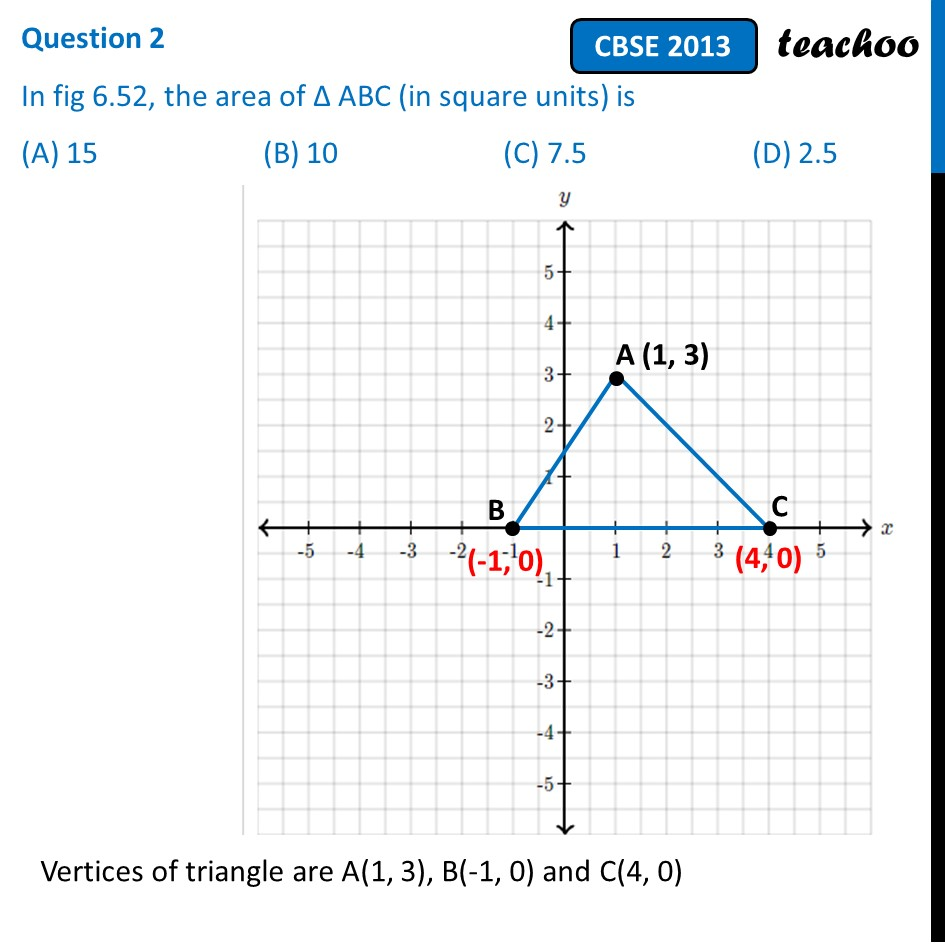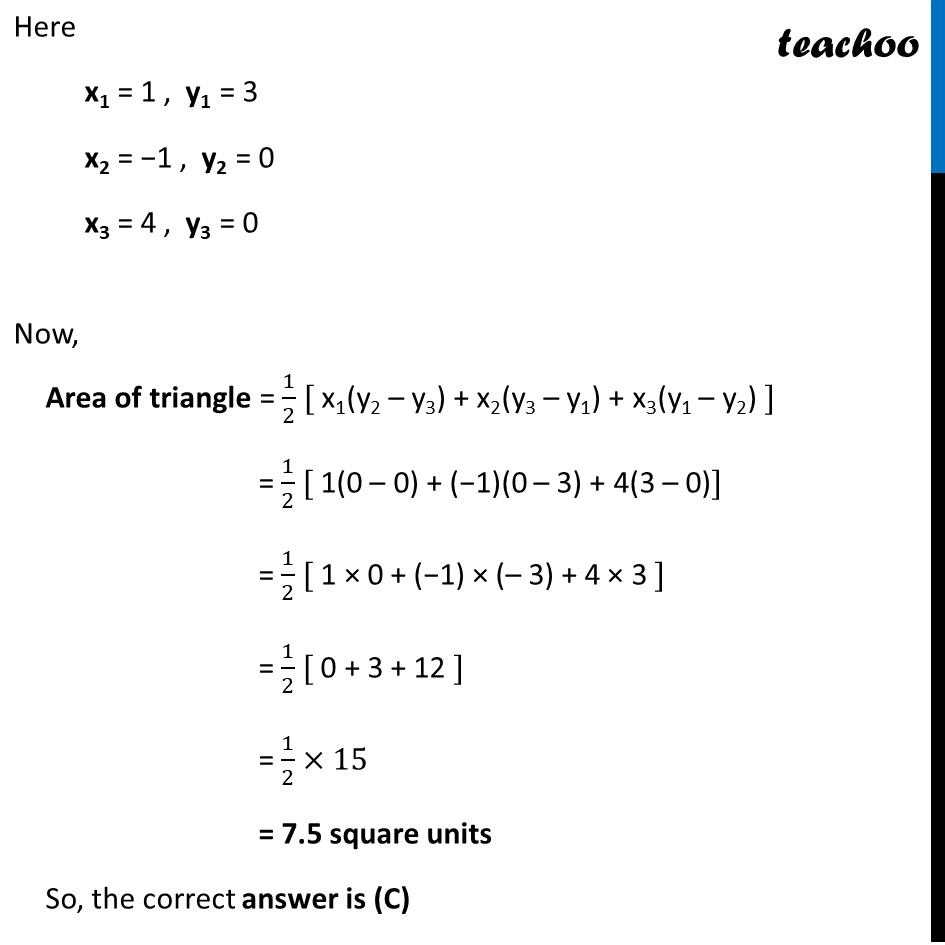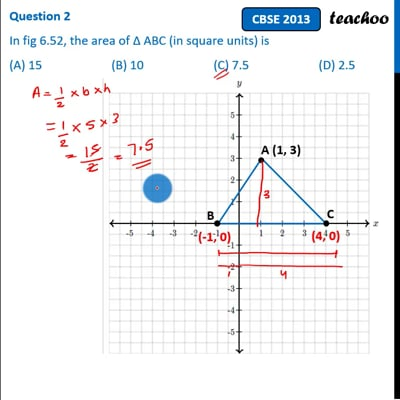Past Year MCQ

Chapter 7 Class 10 Coordinate Geometry (Term 1)
Serial order wise

## (D) 2.5

This question is similar to Example 11 - Chapter 7 Class 10 Coordinate GeometryThis video is only available for Teachoo black users

### Transcript

Question 2 In fig 6.52, the area of ∆ ABC (in square units) is (A) 15 (B) 10 (C) 7.5 (D) 2.5 Vertices of triangle are A(1, 3), B(-1, 0) and C(4, 0) Here x1 = 1 , y1 = 3 x2 = −1 , y2 = 0 x3 = 4 , y3 = 0 Now, Area of triangle = 1/2 [ x1(y2 – y3) + x2(y3 – y1) + x3(y1 – y2) ] = 1/2 [ 1(0 – 0) + (−1)(0 – 3) + 4(3 – 0)] = 1/2 [ 1 × 0 + (−1) × (– 3) + 4 × 3 ] = 1/2 [ 0 + 3 + 12 ] = 1/2 × 15 = 7.5 square units So, the correct answer is (C)